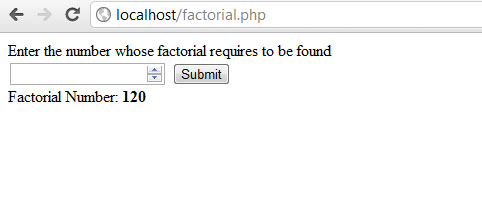# Factorial Number Program in PHP

6/6/2012 code-examplephp

### # Summary

Simple program to get factorial number of any desired number by user. The following code makes use of a function and with the help of loops and other variables returns the calculated Factorial value of the number.

### # Screenshot### # Code

``````<?
/**
*  Function to get Factorial of a Number
*  Rohan Sakhale
*  17th March 2012
*/

function getFactorial(\$num)
{
\$fact = 1;
for(\$i = 1; \$i <= \$num ;\$i++)
\$fact = \$fact * \$i;
return \$fact;
}
?>
<!doctype html>
<html>
<title>Factorial Program in PHP</title>
<body>
<form action="" method="post">
Enter the number whose factorial requires to be found<br />
<input type="number" name="number" id="number" maxlength="4" autofocus required/>
<input type="submit" name="submit" value="Submit" />
</form>
<?
if(isset(\$_POST['submit']) and \$_POST['submit'] == "Submit")
{
if(isset(\$_POST['number']) and is_numeric(\$_POST['number']))
{
echo 'Factorial Number: <strong>'.getFactorial(\$_POST['number']).'</strong>';
}
else
{
echo 'You need to enter number';
}
}

?>
</body>
</html>
``````
1
2
3
4
5
6
7
8
9
10
11
12
13
14
15
16
17
18
19
20
21
22
23
24
25
26
27
28
29
30
31
32
33
34
35
36
37
38
39
40
41
42